# LAMBDA function

You can create and return a custom function with a set of names and a `formula_expression` that uses them. To calculate the `formula_expression`, you can call the returned function with as many values as the `name` declares.

## Sample Usage

`LAMBDA(Salary, Salary*0.3)(1000)`

`LAMBDA(Temp, (5/9)*(Temp-32))(85)`

## Syntax

`LAMBDA(name, formula_expression)`

• `name`: The name to be used inside the `formula_expression`. This name must be an identifier and resolves to the actual value passed to the custom function returned by `LAMBDA`.
• `formula_expression`: The formula to be calculated. It uses names declared in previous parameters.

## Notes

• If a named function expects another function as an input in an argument inside a named function, you can use a `LAMBDA` or a named function.

## Examples

Example data: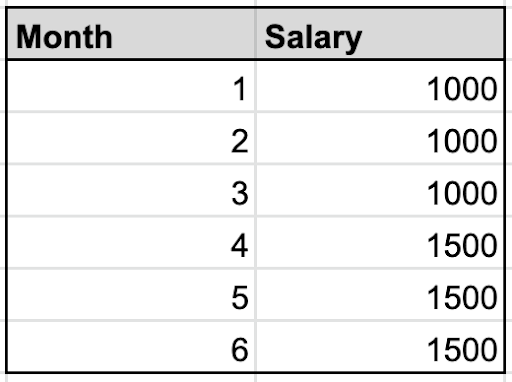### LAMBDA standalone

You can write a `LAMBDA` function to calculate salary tax, assuming that the tax rate is 30%. You input the salary value after the function.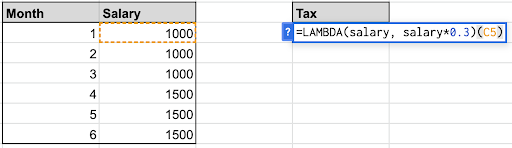Example: `=LAMBDA(salary, salary*0.3)(C5)`

This calculates the tax for month 1.

### LAMBDA inside a LAMBDA helper function

To perform advanced array-operations, use `LAMBDA` functions inside `LAMBDA helper functions` (LHFs).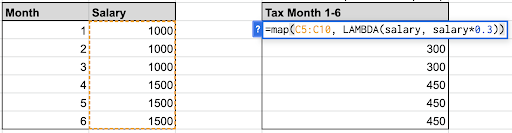Example: `=MAP(C5:C10, LAMBDA(salary, salary*0.3))`

This performs the calculation of salary * 0.3 to each item in the C5:C10 array.

### LAMBDA inside a named function

You can use `LAMBDA` functions as a part of a named function’s argument. Learn more about named functions.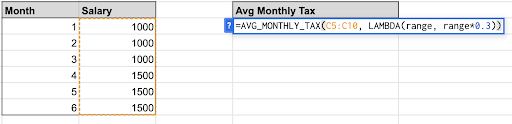Named function: `AVG_MONTHLY_TAX(range, tax_calculator_function)`

Formula definition: `=tax_calculator_function(sum(range))/count(range)`

You can write the `tax_calculator_function` argument with a `LAMBDA` function.

Example: `=AVG_MONTHLY_TAX(C5:C10,LAMBDA(range, range*0.3))`

This calculates the average monthly tax for months 1–6.

## Common errors

### The name argument isn’t an identifier

Example: `=LAMBDA(3, x+1)(3)`

If the first argument isn't an identifier, this error occurs: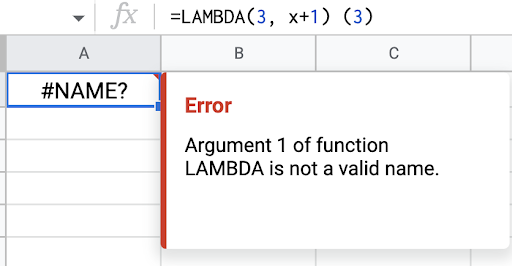Identifier requirements:

• Can’t be ranges, like “A1” or “A2.”
• Can’t have spaces or special characters. Dots and underscores are allowed.

### The LAMBDA wasn’t called

This error is due to not following the `LAMBDA` with the call that contains the values.

Example: `=LAMBDA(salary, salary*0.3)`

If no value is passed for `salary`, this error occurs: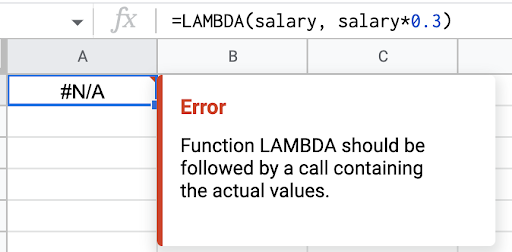For a `LAMBDA`, as an argument in a named function, this means not calling the placeholder referring to that `LAMBDA` with the needed values inside the named function's definition.

Example: Under formula definition, if you write `=tax_calculator_function/count(range)`

instead of `=tax_calculator_function(sum(range))/count(range)`, this error occurs: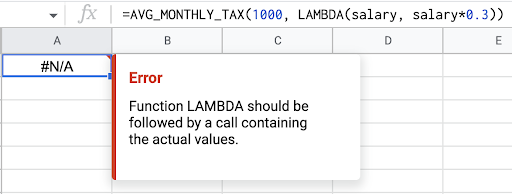Tip: For a` LAMBDA` inside a `LAMBDA helper function`, this error wouldn’t occur because the `LAMBDA helper function` automatically uses the` LAMBDA` on the given input range.

## Lambda helper functions

`Lambda helper functions` (LHFs) are native functions which accept a reusable `LAMBDA` as an argument along with an input array(s). They help in advanced array-operations by executing the formula specified inside the `LAMBDA`, on each value in the input array. The reusable `LAMBDA` can be passed either as a `LAMBDA` function or a `named function`.
Lambda helper functions:

• MAP function: This function maps each value in the given arrays to a new value.
• REDUCE function: This function reduces an array to an accumulated result.
• BYCOL function: This function groups an array by columns.
• BYROW function: This function groups an array by rows.
• SCAN function: This function scans an array and produces intermediate values.
• MAKEARRAY function: This function creates a calculated array of specified dimensions.

## Related functions

Create & use named functions: Let users create and store custom functions, similar to `LAMBDA`.

Search
Clear search
Close search